## Visualizing and Interpreting Convolutional Neural Network

Published: 09 Oct 2015 Category: deep_learning

# Papers

Deconvolutional Networks

Visualizing and Understanding Convolutional Network

Deep Inside Convolutional Networks: Visualising Image Classification Models and Saliency Maps

Understanding Deep Image Representations by Inverting Them

deepViz: Visualizing Convolutional Neural Networks for Image Classification

Inverting Convolutional Networks with Convolutional Networks

Understanding Neural Networks Through Deep Visualization

Visualizing Higher-Layer Features of a Deep Network

Generative Modeling of Convolutional Neural Networks

Understanding Intra-Class Knowledge Inside CNN

Learning FRAME Models Using CNN Filters for Knowledge Visualization

Convergent Learning: Do different neural networks learn the same representations?

Visualizing and Understanding Deep Texture Representations

Visualizing Deep Convolutional Neural Networks Using Natural Pre-Images

An Interactive Node-Link Visualization of Convolutional Neural Networks

Learning Deep Features for Discriminative LocalizationMultifaceted Feature Visualization: Uncovering the Different Types of Features Learned By Each Neuron in Deep Neural NetworksA New Method to Visualize Deep Neural Networks

A Taxonomy and Library for Visualizing Learned Features in Convolutional Neural Networks

VisualBackProp: visualizing CNNs for autonomous driving

VisualBackProp: efficient visualization of CNNs

Grad-CAM: Why did you say that? Visual Explanations from Deep Networks via Gradient-based LocalizationGrad-CAM: Why did you say that?

Visualizing Residual Networks

Visualizing Deep Neural Network Decisions: Prediction Difference Analysis

ActiVis: Visual Exploration of Industry-Scale Deep Neural Network Models

Picasso: A Neural Network Visualizer

CNN Fixations: An unraveling approach to visualize the discriminative image regions

A Forward-Backward Approach for Visualizing Information Flow in Deep Networks

Using KL-divergence to focus Deep Visual Explanation

https://arxiv.org/abs/1711.06431

An Introduction to Deep Visual Explanation

Visual Explanation by Interpretation: Improving Visual Feedback Capabilities of Deep Neural Networks

https://arxiv.org/abs/1712.06302

Visualizing the Loss Landscape of Neural Nets

Visualizing Deep Similarity Networks

https://arxiv.org/abs/1901.00536

## Interpreting Convolutional Neural Networks

Network Dissection: Quantifying Interpretability of Deep Visual Representations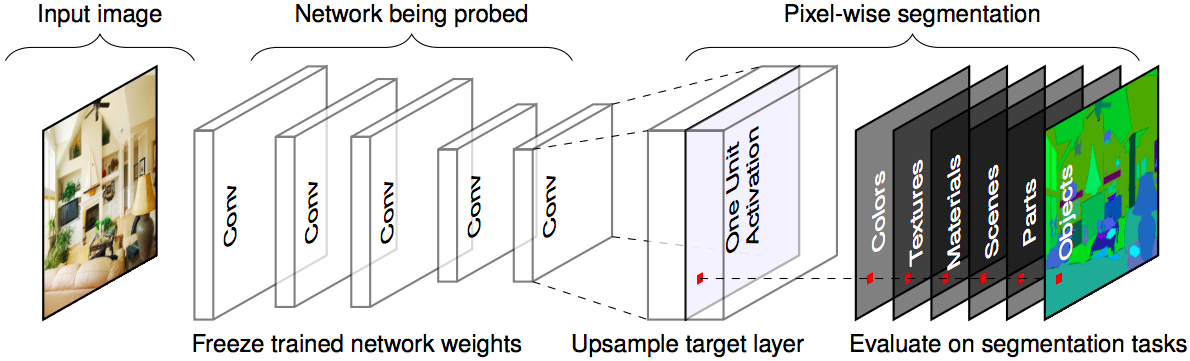Interpreting Deep Visual Representations via Network Dissection

https://arxiv.org/abs/1711.05611

Methods for Interpreting and Understanding Deep Neural Networks

SVCCA: Singular Vector Canonical Correlation Analysis for Deep Learning Dynamics and Interpretability

Towards Interpretable Deep Neural Networks by Leveraging Adversarial Examples

Interpretable Convolutional Neural Networks

https://arxiv.org/abs/1710.00935

Interpreting Convolutional Neural Networks Through Compression

Interpreting Deep Neural Networks

Interpreting CNNs via Decision Trees

https://arxiv.org/abs/1802.00121

Visual Interpretability for Deep Learning: a Survey

https://arxiv.org/abs/1802.00614

Interpreting Deep Classifier by Visual Distillation of Dark Knowledge

How convolutional neural network see the world - A survey of convolutional neural network visualization methods

Understanding Regularization to Visualize Convolutional Neural Networks

Deeper Interpretability of Deep Networks

Interpretable CNNs

https://arxiv.org/abs/1901.02413

Explaining AlphaGo: Interpreting Contextual Effects in Neural Networks

https://arxiv.org/abs/1901.02184

Interpretable BoW Networks for Adversarial Example Detection

https://arxiv.org/abs/1901.02229

Deep Features Analysis with Attention Networks

Understanding Neural Networks via Feature Visualization: A survey

Explaining Neural Networks via Perturbing Important Learned Features

https://arxiv.org/abs/1911.11081

Interpreting Adversarially Trained Convolutional Neural Networks

# Projects

Interactive Deep Neural Net Hallucinations

torch-visbox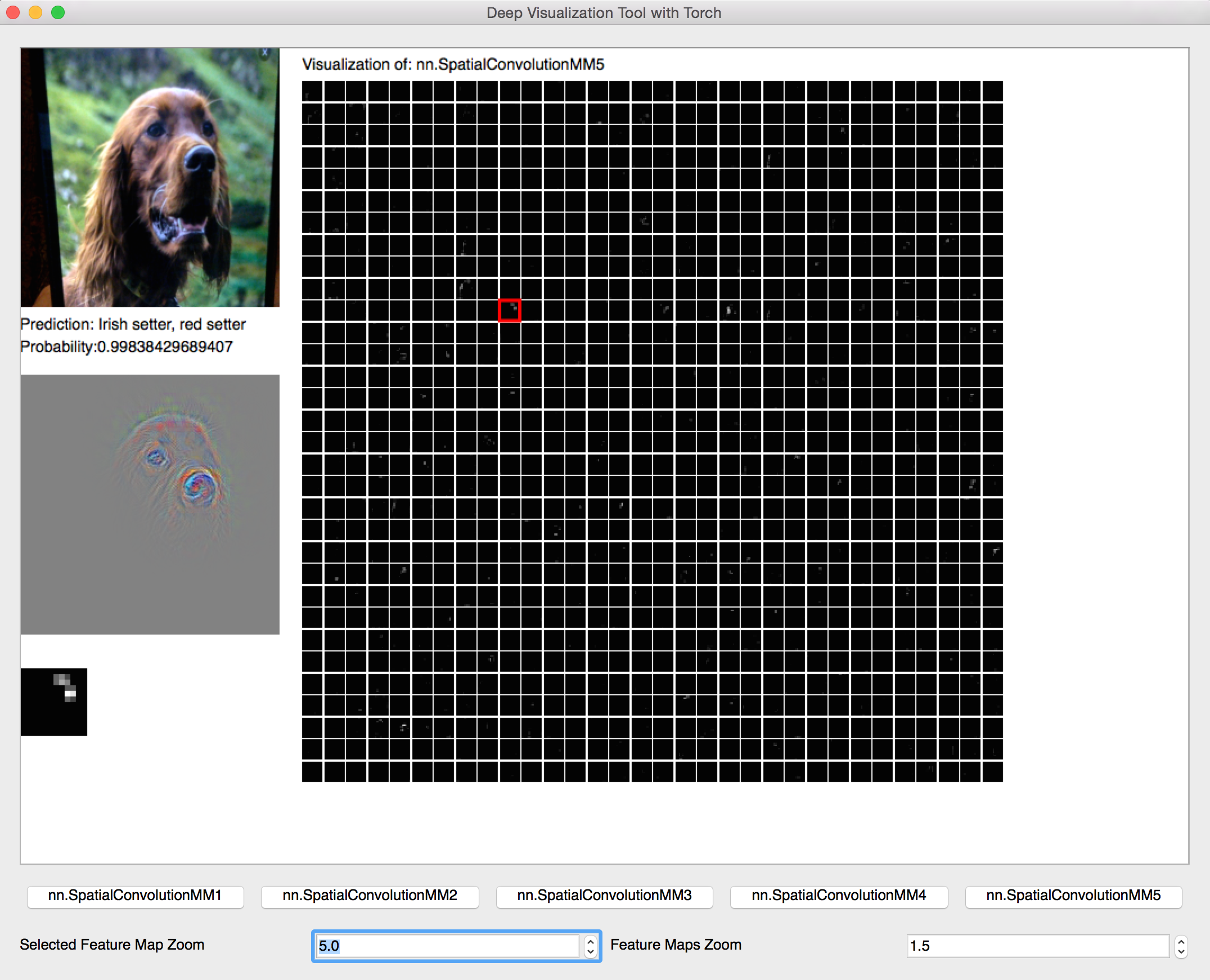draw_convnet: Python script for illustrating Convolutional Neural Network (ConvNet)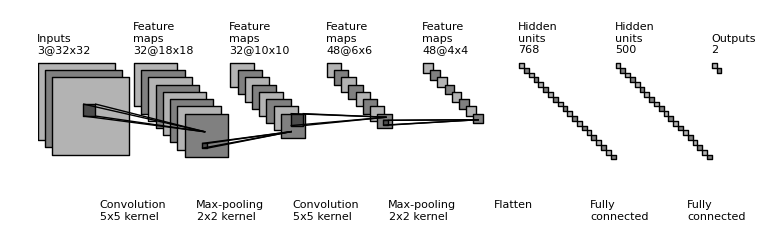Caffe prototxt visualization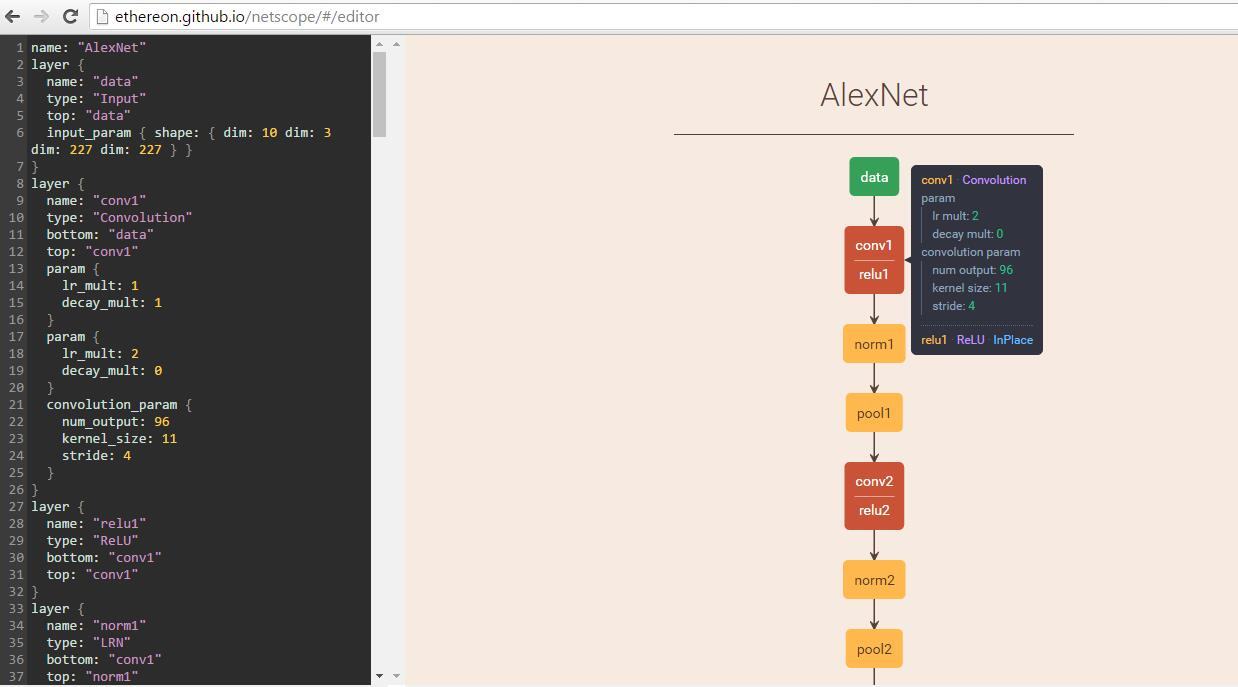Keras Visualization Toolkit

mNeuron: A Matlab Plugin to Visualize Neurons from Deep Models

cnnvis-pytorch

VisualDL

# Blogs

Visualizing CNN architectures side by side with mxnetHow convolutional neural networks see the world: An exploration of convnet filters with Keras

Visualizing Deep Learning with t-SNE (Tutorial and Video)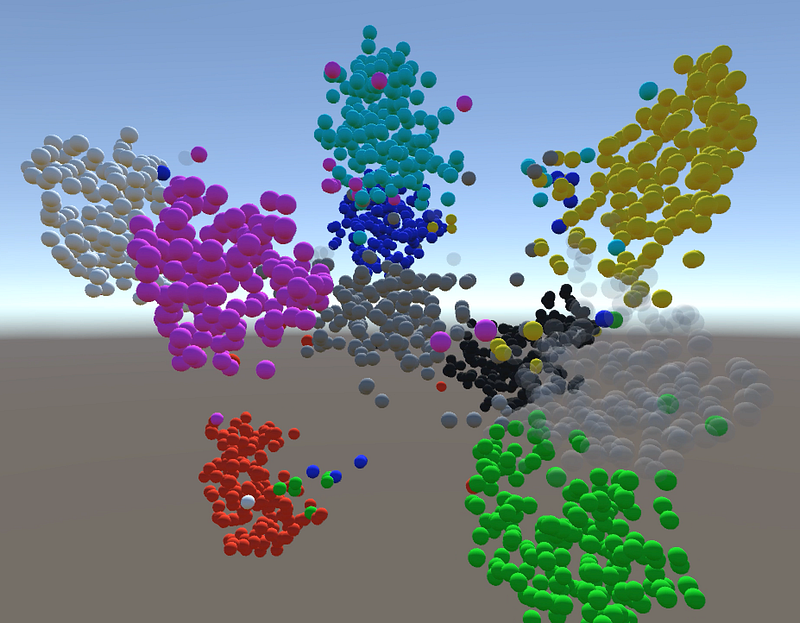Peeking inside Convnets

Visualizing Features from a Convolutional Neural Network

Visualizing Deep Neural Networks Classes and Features

http://ankivil.com/visualizing-deep-neural-networks-classes-and-features/

Visualizing parts of Convolutional Neural Networks using Keras and Cats

Visualizing convolutional neural networks

# Tools

Topological Visualisation of a Convolutional Neural Network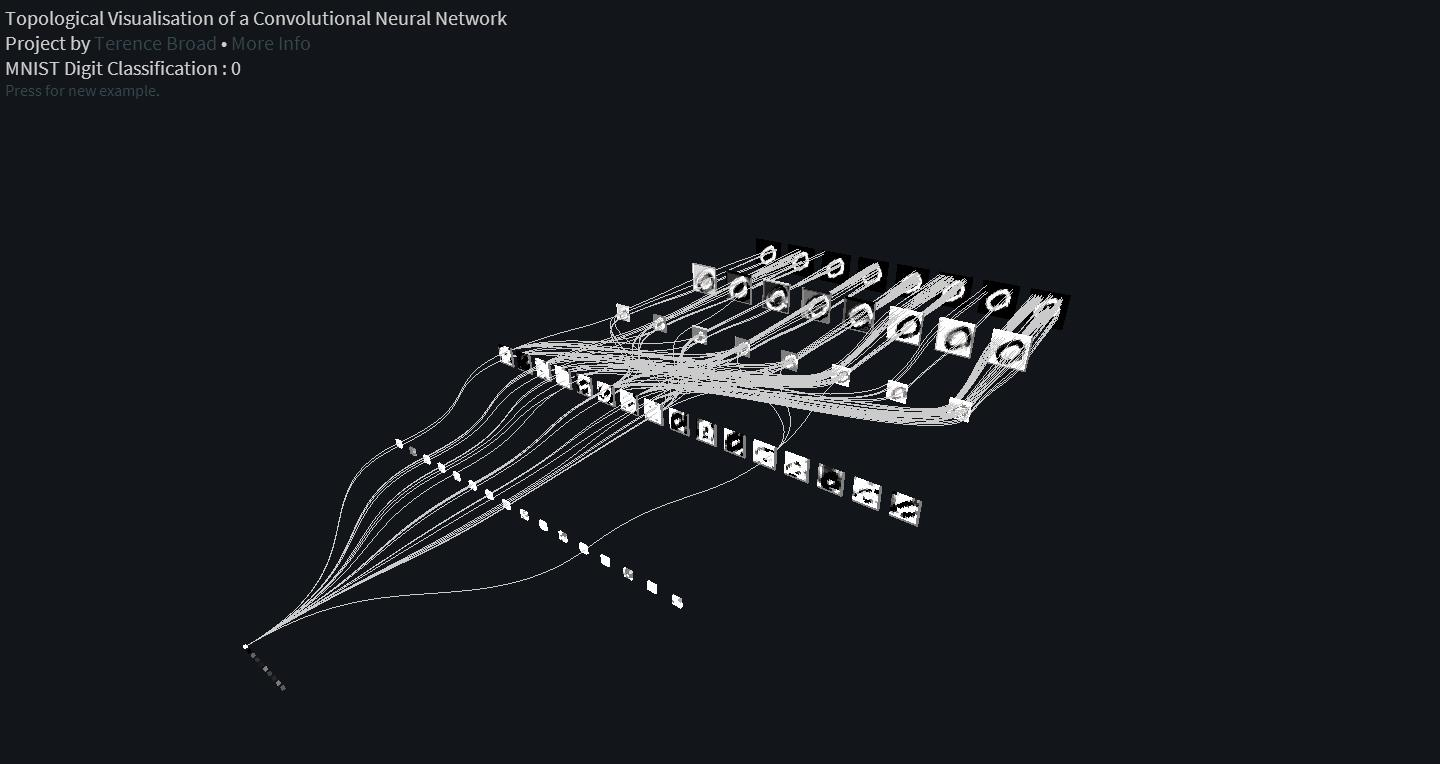Visualization of Places-CNN and ImageNet CNN

Visualization of a feed forward Neural Network using MNIST dataset

CNNVis: Towards Better Analysis of Deep Convolutional Neural Networks.

http://shixialiu.com/publications/cnnvis/demo/

Quiver: Interactive convnet features visualization for Keras

Netron